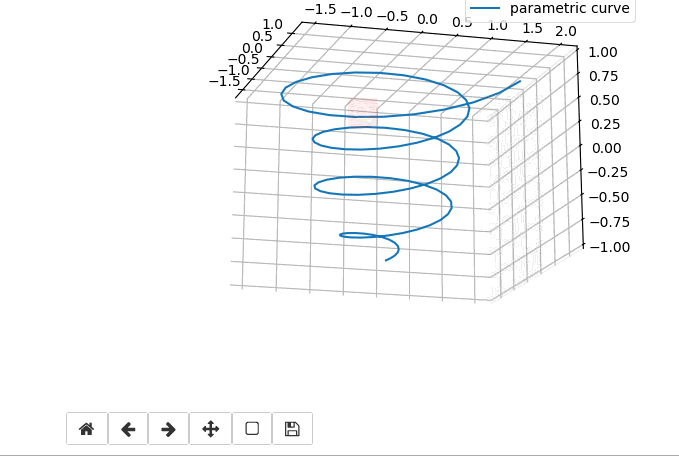# Home button is useless in Jupyter Notebook

Hi all,
I was using matplotlib to plot my 3D curve on Jupyter Notebook today, and I rotated the image with my mouse,
And I want to use the Home button in the Interactive toobar, which says Reset original view.
However, it did not work, and I do not know if I had write the right code,
and here is the code:
%matplotlib notebook
# -- coding: UTF-8 --
import matplotlib as mpl
from mpl_toolkits.mplot3d import Axes3D
import numpy as np
import matplotlib.pyplot as plt

``````mpl.rcParams['legend.fontsize'] = 10
fig = plt.figure()

ax = fig.gca(projection='3d')

theta = np.linspace(-4 * np.pi, 4 * np.pi, 100)
z = np.linspace(-4, 4, 100) / 4
r = z**3 + 1
x = r * np.sin(theta)
y = r * np.cos(theta)

ax.plot(x, y, z, label='parametric curve')

ax.legend()

plt.show()
``````

And here is the screenshot of the curve I have rotated with my mouse,See, it did not resume to the initial view as started, after I had clicked the Home button.

I think it very strange and I do not know how to fix it.

Any suggestions would be appreciated.

Kind regards,
Eric